Search by Topic

Resources tagged with Interactivities similar to Complex Sine:

Filter by: Content type:
Age range:
Challenge level:

There are 74 results

Broad Topics > Information and Communications Technology > InteractivitiesPower Match

Age 16 to 18 Challenge Level:

Can you locate these values on this interactive logarithmic scale?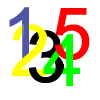Complex Countdown

Age 16 to 18 Challenge Level:

Play a more cerebral countdown using complex numbers.Tessellation Interactivity

Age 7 to 16 Challenge Level:

An environment that enables you to investigate tessellations of regular polygonsInteractive Workout - Mathmo

Age 16 to 18 Short Challenge Level:

Mathmo is a revision tool for post-16 mathematics. It's great installed as a smartphone app, but it works well in pads and desktops and notebooks too. Give yourself a mathematical workout!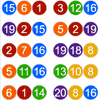Lottery Simulator

Age 7 to 16 Challenge Level:

Use this animation to experiment with lotteries. Choose how many balls to match, how many are in the carousel, and how many draws to make at once.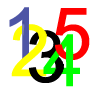Matrix Countdown

Age 16 to 18 Challenge Level:

Play countdown with matricesNapoleon's Theorem

Age 14 to 18 Challenge Level:

Triangle ABC has equilateral triangles drawn on its edges. Points P, Q and R are the centres of the equilateral triangles. What can you prove about the triangle PQR?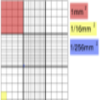Using the Haemocytometer

Age 14 to 16 Challenge Level:

Practise your skills of proportional reasoning with this interactive haemocytometer.Mixed up Mixture

Age 14 to 16 Challenge Level:

Can you fill in the mixed up numbers in this dilution calculation?A Question of Scale

Age 14 to 16 Challenge Level:

Use your skill and knowledge to place various scientific lengths in order of size. Can you judge the length of objects with sizes ranging from 1 Angstrom to 1 million km with no wrong attempts?Investigating the Dilution Series

Age 14 to 16 Challenge Level:

Which dilutions can you make using only 10ml pipettes?Inside Out

Age 14 to 16 Challenge Level:

There are 27 small cubes in a 3 x 3 x 3 cube, 54 faces being visible at any one time. Is it possible to reorganise these cubes so that by dipping the large cube into a pot of paint three times you. . . .Cushion Ball Interactivity

Age 14 to 16 Challenge Level:

The interactive diagram has two labelled points, A and B. It is designed to be used with the problem "Cushion Ball"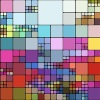Squaring the Rectangle

Age 14 to 18 Challenge Level:

Can you find a way to turn a rectangle into a square?Excel Interactive Resource: Interactive Division

Age 11 to 16 Challenge Level:

Use an Excel to investigate division. Explore the relationships between the process elements using an interactive spreadsheet.Countdown Fractions

Age 11 to 16 Challenge Level:

Here is a chance to play a fractions version of the classic Countdown Game.30-60-90 Polypuzzle

Age 16 to 18 Challenge Level:

Re-arrange the pieces of the puzzle to form a rectangle and then to form an equilateral triangle. Calculate the angles and lengths.Attractive Tablecloths

Age 14 to 16 Challenge Level:

Charlie likes tablecloths that use as many colours as possible, but insists that his tablecloths have some symmetry. Can you work out how many colours he needs for different tablecloth designs?Spinners Environment

Age 5 to 18 Challenge Level:

A tool for generating random integers.Loci Resources

Age 14 to 16 Challenge Level:

This set of resources for teachers offers interactive environments to support work on loci at Key Stage 4.Proof Sorter - the Square Root of 2 Is Irrational

Age 16 to 18 Challenge Level:

Try this interactivity to familiarise yourself with the proof that the square root of 2 is irrational. Sort the steps of the proof into the correct order.Spot the Card

Age 14 to 16 Challenge Level:

It is possible to identify a particular card out of a pack of 15 with the use of some mathematical reasoning. What is this reasoning and can it be applied to other numbers of cards?Excel Interactive Resource: Fraction Multiplication

Age 11 to 16 Challenge Level:

Use Excel to explore multiplication of fractions.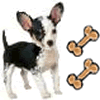Ratio Pairs 3

Age 11 to 16 Challenge Level:

Match pairs of cards so that they have equivalent ratios.Changing Places

Age 14 to 16 Challenge Level:

Place a red counter in the top left corner of a 4x4 array, which is covered by 14 other smaller counters, leaving a gap in the bottom right hand corner (HOME). What is the smallest number of moves. . . .Excel Interactive Resource: Fraction Addition & Fraction Subtraction

Age 11 to 16 Challenge Level:

Use Excel to practise adding and subtracting fractions.Cushion Ball

Age 16 to 18 Challenge Level:

The shortest path between any two points on a snooker table is the straight line between them but what if the ball must bounce off one wall, or 2 walls, or 3 walls?Napoleon's Hat

Age 16 to 18 Challenge Level:

Three equilateral triangles ABC, AYX and XZB are drawn with the point X a moveable point on AB. The points P, Q and R are the centres of the three triangles. What can you say about triangle PQR?Red or Black Spinner

Age 3 to 16 Challenge Level:

A simple spinner that is equally likely to land on Red or Black. Useful if tossing a coin, dropping it, and rummaging about on the floor have lost their appeal. Needs a modern browser; if IE then at. . . .Trigonometric Protractor

Age 14 to 16 Challenge Level:

An environment that simulates a protractor carrying a right- angled triangle of unit hypotenuse.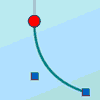Graphical Interpretation

Age 14 to 16 Challenge Level:

This set of resources for teachers offers interactive environments to support work on graphical interpretation at Key Stage 4.Solving with Euclid's Algorithm

Age 14 to 18 Challenge Level:

A java applet that takes you through the steps needed to solve a Diophantine equation of the form Px+Qy=1 using Euclid's algorithm.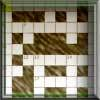Crossword 1

Age 16 to 18 Challenge Level:

A mathematically themed crossword.Excel Interactive Resource: Haitch

Age 11 to 16 Challenge Level:

An Excel spreadsheet with an investigation.Excel Interactive Resource: Multiples Chain

Age 11 to 16 Challenge Level:

Use an interactive Excel spreadsheet to investigate factors and multiples.Excel Interactive Resource: Long Multiplication

Age 11 to 16 Challenge Level:

Use an Excel spreadsheet to explore long multiplication.Excel Interactive Resource: Equivalent Fraction Bars

Age 11 to 16 Challenge Level:

A simple file for the Interactive whiteboard or PC screen, demonstrating equivalent fractions.Excel Interactive Resource: the up and Down Game

Age 11 to 16 Challenge Level:

Use an interactive Excel spreadsheet to explore number in this exciting game!Excel Interactive Resource: Number Grid Functions

Age 11 to 16 Challenge Level:

Use Excel to investigate the effect of translations around a number grid.Percentages for Key Stage 4

Age 14 to 16 Challenge Level:

A group of interactive resources to support work on percentages Key Stage 4.Factors and Multiples - Secondary Resources

Age 11 to 16 Challenge Level:

A collection of resources to support work on Factors and Multiples at Secondary level.White Box

Age 7 to 18 Challenge Level:

This game challenges you to locate hidden triangles in The White Box by firing rays and observing where the rays exit the Box.Direct Logic

Age 16 to 18 Challenge Level:

Can you work through these direct proofs, using our interactive proof sorters?Interactive Number Patterns

Age 14 to 16 Challenge Level:

How good are you at finding the formula for a number pattern ?Fractions and Percentages Card Game

Age 11 to 16 Challenge Level:

Match the cards of the same value.Cyclic Triangles

Age 16 to 18 Challenge Level:

Make and prove a conjecture about the cyclic quadrilateral inscribed in a circle of radius r that has the maximum perimeter and the maximum area.Got a Strategy for Last Biscuit?

Age 11 to 16 Challenge Level:

Can you beat the computer in the challenging strategy game?Square It

Age 11 to 16 Challenge Level:

Players take it in turns to choose a dot on the grid. The winner is the first to have four dots that can be joined to form a square.A Tilted Square

Age 14 to 16 Challenge Level:

The opposite vertices of a square have coordinates (a,b) and (c,d). What are the coordinates of the other vertices?Guesswork

Age 14 to 16 Challenge Level:

Ask a friend to choose a number between 1 and 63. By identifying which of the six cards contains the number they are thinking of it is easy to tell them what the number is.